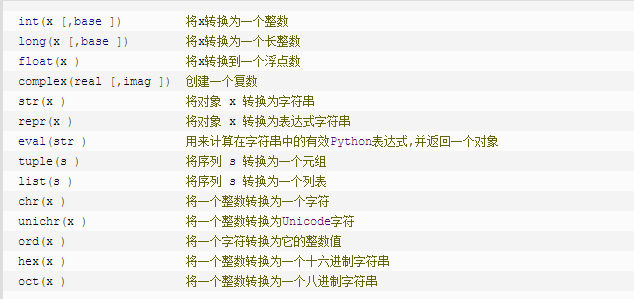## 1、Python数学函数

1、abs(x)：取绝对值，内建函数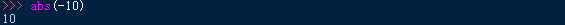2、math.ceil(x)：向上取整，在math模块中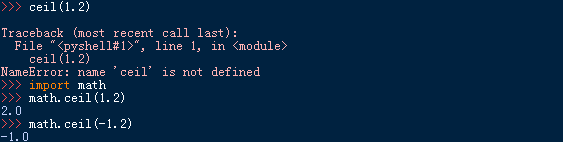3、cmp(x,y)：如果 x < y ，返回-1；如果 x == y ，返回0；如果 x > y ，返回1。内建函数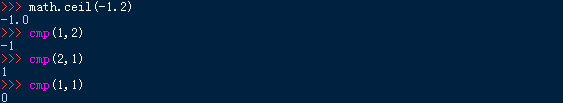4、math.exp(x)：在math模块中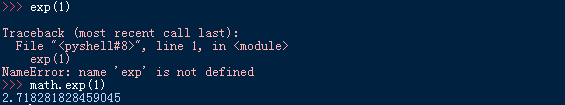5、math.fabs(x)：返回数字的绝对值，在math模块中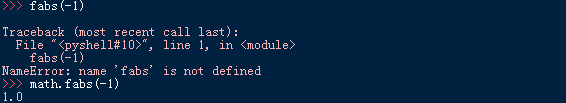6、math.floor(x)：向下取整，在math模块中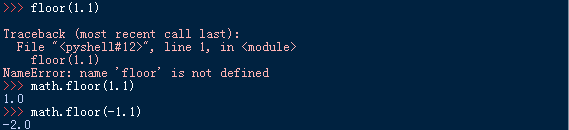7、math.log10(x)：返回以10为底数的x的对数，在math模块中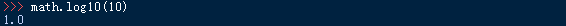8、math.e    math.pi

9、math.log(x)：返回以e为底数的x的对数，在math模块中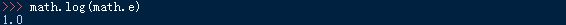10、max(x,y,z)或max(list)11、min(x,y,z)或min(list)

12、math.modf(x)：返回x的小数部分和整数部分，两部分符号和x的符号相同，且整数部分用浮点型表示。在math模块中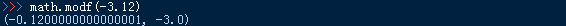注意：小数部分不是精确的！！！

13、pow(x,y)：乘方，内建函数，等价于**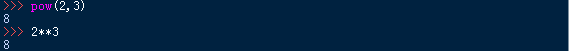14、round(x)：四舍五入，内建函数15、math.sqrt(x)：在math模块中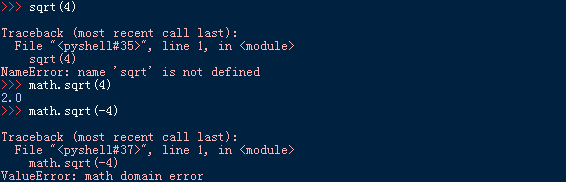16、cmath.sqrt(x)：在cmath模块中## 2、Python随机数函数

1、random.choice(list)：返回一个序列（列表，元组或字符串）的随机项，在random模块中2、random.randrange([start,]stop[,step])：返回指定递增基数集合中的一个随机数，基数缺省值为1，在random模块中

start：指定范围内的开始值，包含在范围内

stop：指定范围内的结束值，不包含在范围内

step：指定递增基数3、random.random()：随机生成一个在[0,1)中的实数，在random模块中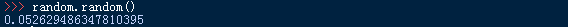4、random.seed([x])：改变随机数生成器的种子，可以在调用其他随机模块函数前调用此函数

x — 改变随机数生成器的种子seed。如果你不了解其原理，你不必特别去设定seed，Python会帮你选择seed。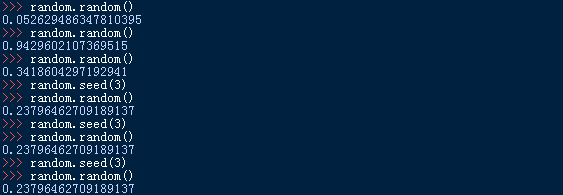5、random.shuffle(lst)：将列表的所有元素随机排序，返回排序后的列表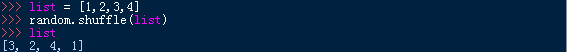6、random.uniform(x,y)：随机生成一个在[x,y]中的实数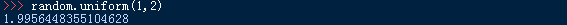## 3、Python三角函数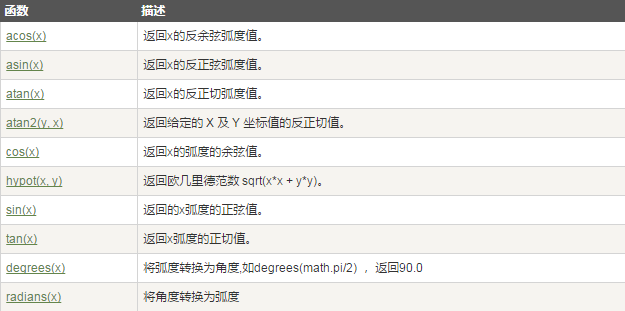## 4、Python常量值

自然对数：math.e
圆周率：math.pi

## 5、Python数值类型转换